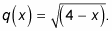##### Trigonometry Workbook For DummiesCertain mathematical expressions allow you to combine stretching, shrinking, translating, and reflecting a function all into one graph. An expression that shows all the transformations in one is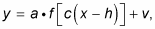where

• a is the vertical transformation.

• c is the horizontal transformation.

• h is the horizontal shift.

• v is the vertical shift.

For instance, f(x) = –2(x – 1)2 + 4 moves the graph of y = x2 right 1 unit, vertically stretches it by a factor of 2, reflects it upside down, and then moves it up 4 units.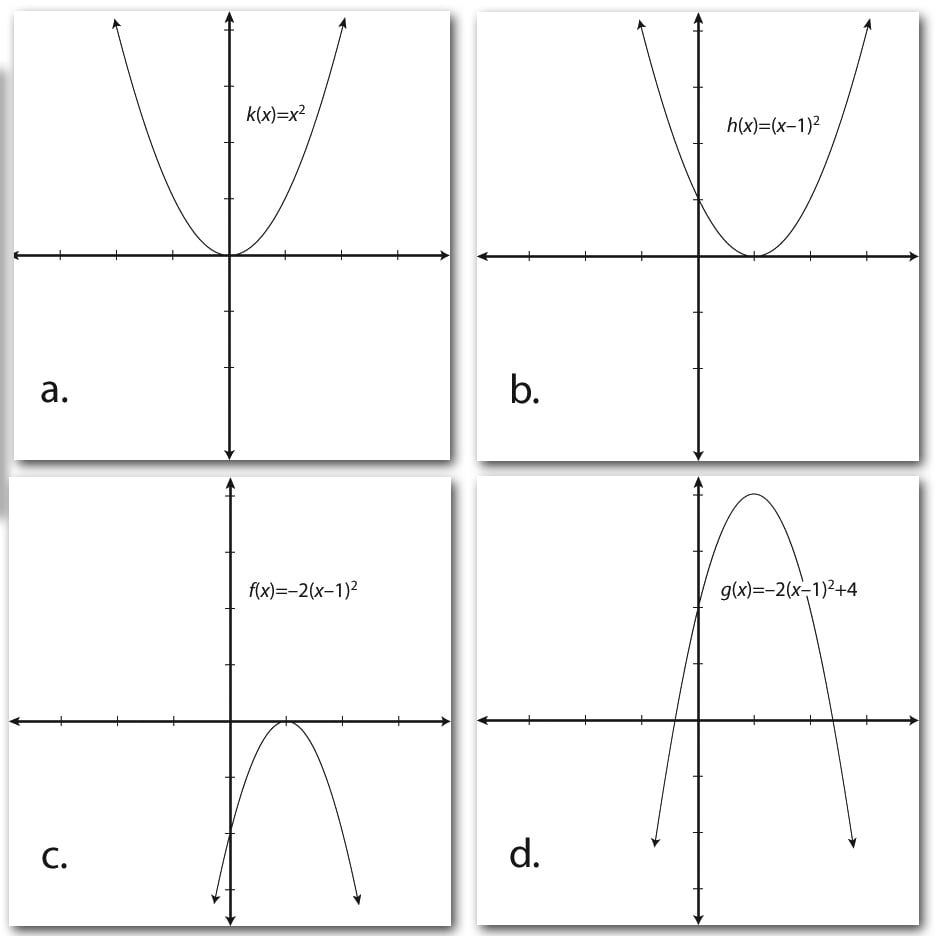This figure shows each stage.

• Figure a is the parent graph: k(x) = x2.

• Figure b is the horizontal shift to the right by one: h(x) = (x – 1)2.

• Figure c is the vertical stretch of two: f(x) = –2(x – 1)2. (Notice that because the value was negative, the graph was also turned upside down.)

• Figure d is the vertical shift up by four: g(x) = –2(x – 1)2 + 4.

The following transformation illustrates the importance of the order of the process. You graph the function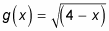with the following steps:

1. Rewrite the function in the form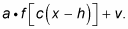Reorder the function so that the x comes first (in descending order). And don’t forget the negative sign! Here it is: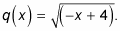2. Factor out the coefficient in front of the x.

You now have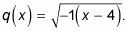3. Reflect the parent graph.

Because the –1 is inside the square-root function, q(x) is a horizontal reflection over a vertical line of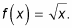4. Shift the graph.

The factored form of q(x) (from Step 2) reveals that the horizontal shift is four to the right.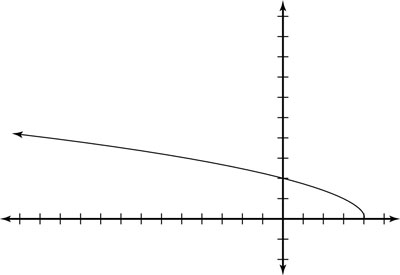This figure shows the graph of